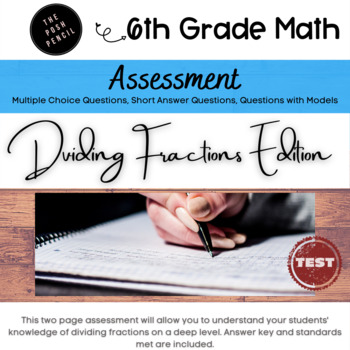# Dividing Fractions 5th/6th Grade AssessmentSubject
Resource Type
File Type

PDF

(858 KB|5 pages)
Product Rating
Standards
Also included in:
1. This Dividing Fractions Study Guide and Assessment resource has all you need to help your students prepare and assess their knowledge of the common core standards related to dividing fractions in sixth grade.The study guide mimics the assessment so that your students will be familiar with the types
\$3.00
\$2.70
Save \$0.30
• Product Description
• StandardsNEW

This Dividing Fractions Assessment is a 9 question assessment that will allow you to review your students' knowledge of many state standards related to dividing fractions.

The assessment includes:

• multiple choice questions
• equations that require work to be shown
• equations with models

Included with this assessment is an answer key and featured on the printable assessment are growth mindset messages to keep your students motivated while they are taking their assessment!

If you are looking for an accompanying Study Guide, here you go!

Or buy as a bundle and save!

Thank you, and enjoy!

Permission to copy for single classroom use only.

Electronic distribution limited to single classroom use only. Not for public display.

Interpret and compute quotients of fractions, and solve word problems involving division of fractions by fractions, e.g., by using visual fraction models and equations to represent the problem. For example, create a story context for (2/3) ÷ (3/4) and use a visual fraction model to show the quotient; use the relationship between multiplication and division to explain that (2/3) ÷ (3/4) = 8/9 because 3/4 of 8/9 is 2/3. (In general, (𝘢/𝘣) ÷ (𝘤/𝘥) = 𝘢𝘥/𝘣𝘤.) How much chocolate will each person get if 3 people share 1/2 lb of chocolate equally? How many 3/4-cup servings are in 2/3 of a cup of yogurt? How wide is a rectangular strip of land with length 3/4 mi and area 1/2 square mi?
Solve real world problems involving division of unit fractions by non-zero whole numbers and division of whole numbers by unit fractions, e.g., by using visual fraction models and equations to represent the problem. For example, how much chocolate will each person get if 3 people share 1/2 lb of chocolate equally? How many 1/3-cup servings are in 2 cups of raisins?
Interpret division of a whole number by a unit fraction, and compute such quotients. For example, create a story context for 4 ÷ (1/5), and use a visual fraction model to show the quotient. Use the relationship between multiplication and division to explain that 4 ÷ (1/5) = 20 because 20 × (1/5) = 4.
Interpret division of a unit fraction by a non-zero whole number, and compute such quotients. For example, create a story context for (1/3) ÷ 4, and use a visual fraction model to show the quotient. Use the relationship between multiplication and division to explain that (1/3) ÷ 4 = 1/12 because (1/12) × 4 = 1/3.
Apply and extend previous understandings of division to divide unit fractions by whole numbers and whole numbers by unit fractions.
Total Pages
5 pages
Included
Teaching Duration
90 minutes
Report this Resource to TpT
Reported resources will be reviewed by our team. Report this resource to let us know if this resource violates TpT’s content guidelines.